# Angle Sum and Difference Identities

Recently whilst revisiting trigonometry I came across a neat way of proving the angle sum and difference identities shown below:

$sin(\alpha+\beta)=sin(\alpha)cos(\beta)+cos(\alpha)sin(\beta)$
$cos(\alpha+\beta)=cos(\alpha)cos(\beta)-sin(\alpha)sin(\beta)$

I have used these formulas a number of times in the past to solve problems but I had not thought much about how they came to be. It was not until I learnt the visual proof I will describe on this post that I finally acquired a solid intuition about what these equations mean.

## Definitions

We will only need two of the right-angled triangle definitions for this proof to work: sine and cosine of an angle.

Consider the right-angled triangle shown below:From the image we can derive the two definitions:

1. $sin(\theta) = \dfrac{opp}{hyp}$ or written in terms of the opposite side: $opp = hyp \cdot sin(\theta)$
2. $cos(\theta) = \dfrac{adj}{hyp}$ or written in terms of the adjacent side: $add = hyp \cdot cos(\theta)$

## Step-by-step visual proof

### Step 1

Draw a rectangle with a right triangle inside as shown in the image.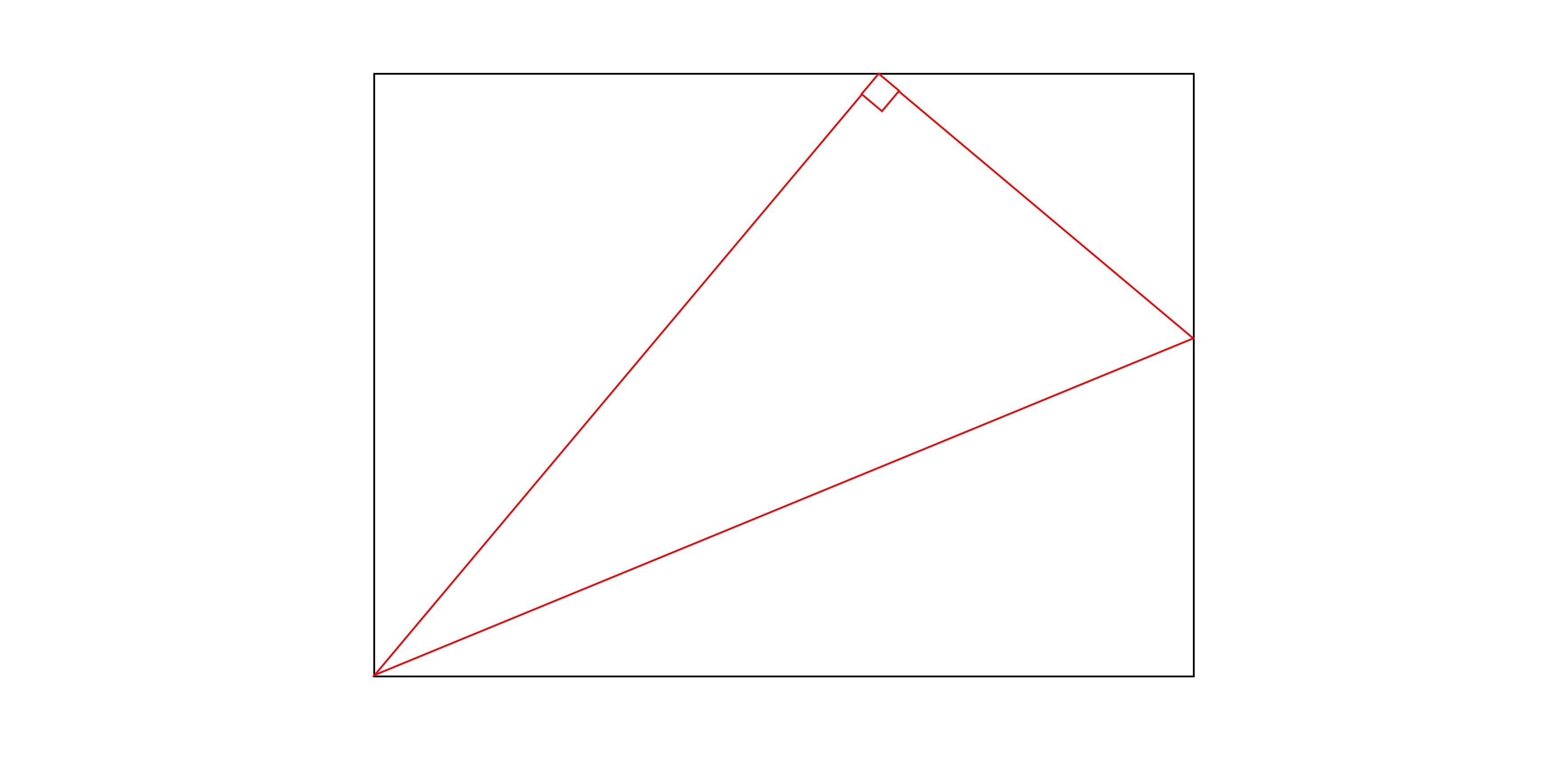### Step 2

Assume the hypotenuse of the triangle is of length 1. We name the angle on the bottom left as $\alpha$ and work out the lengths of the opposite and adjacent sides of the triangle using the definitions.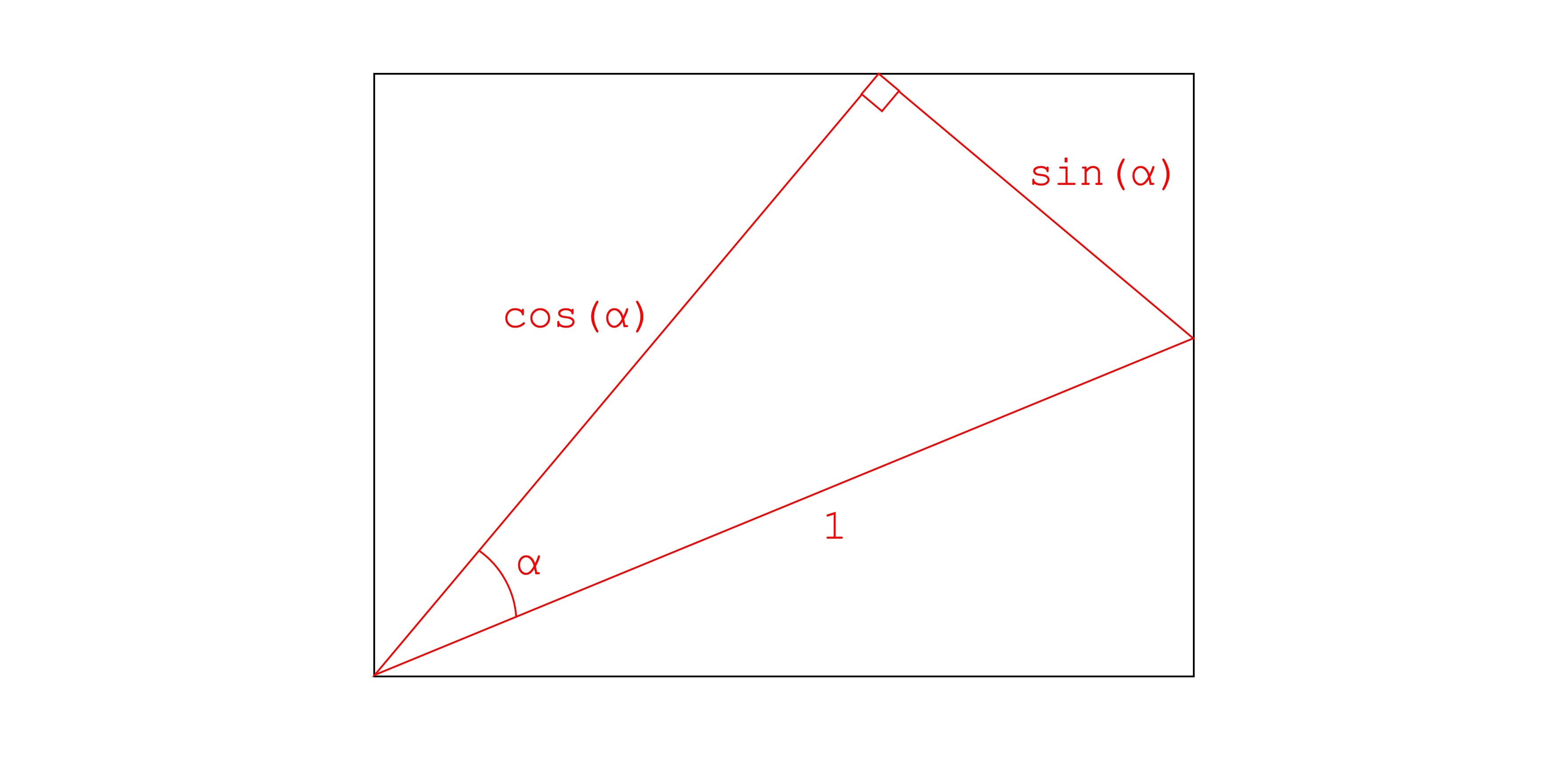### Step 3

We now shift our attention to the triangle at the top left of the rectangle. We name the angle on the bottom left as $\beta$ and use the definitions to find the length of the opposite and adjacent sides of the triangle, having a hypotenuse of length $cos(\alpha)$.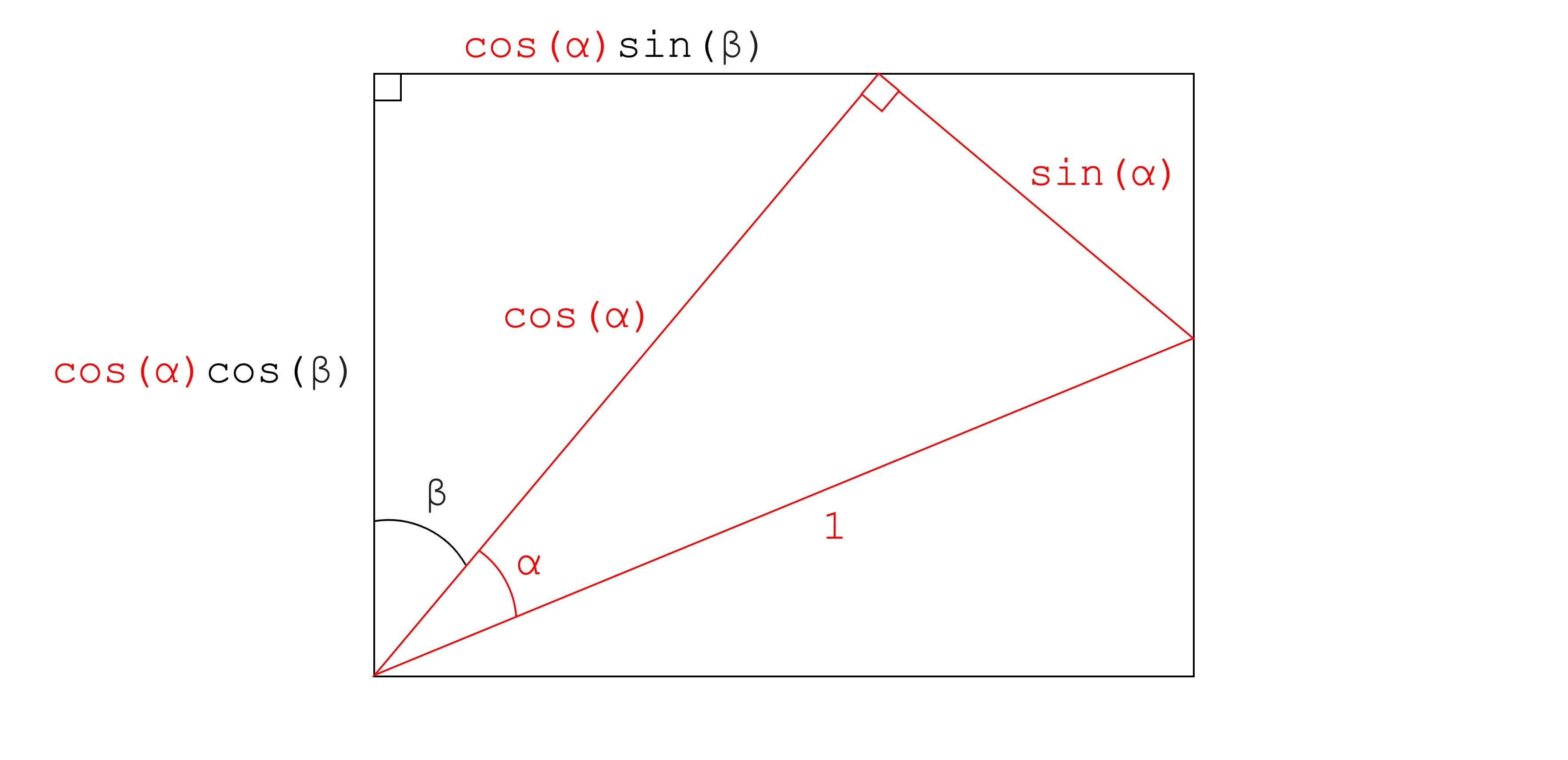### Step 4

To find the length of the sides of the triangle in the top right, we need to use the angles we currently know and the properties of right triangles. If we focus our attention on the corner of the red triangle touching the top of the rectangle, we can see that we have 3 angles that add up to 180°.

We can find the angle on the left by computing $180°-90°-\beta = 90°-\beta$. Then we have a $90°$ angle from the red triangle. And finally, as all these angles add up to 180° we can find the angle on the right by computing $180°-(90°-\beta)-90°=\beta$.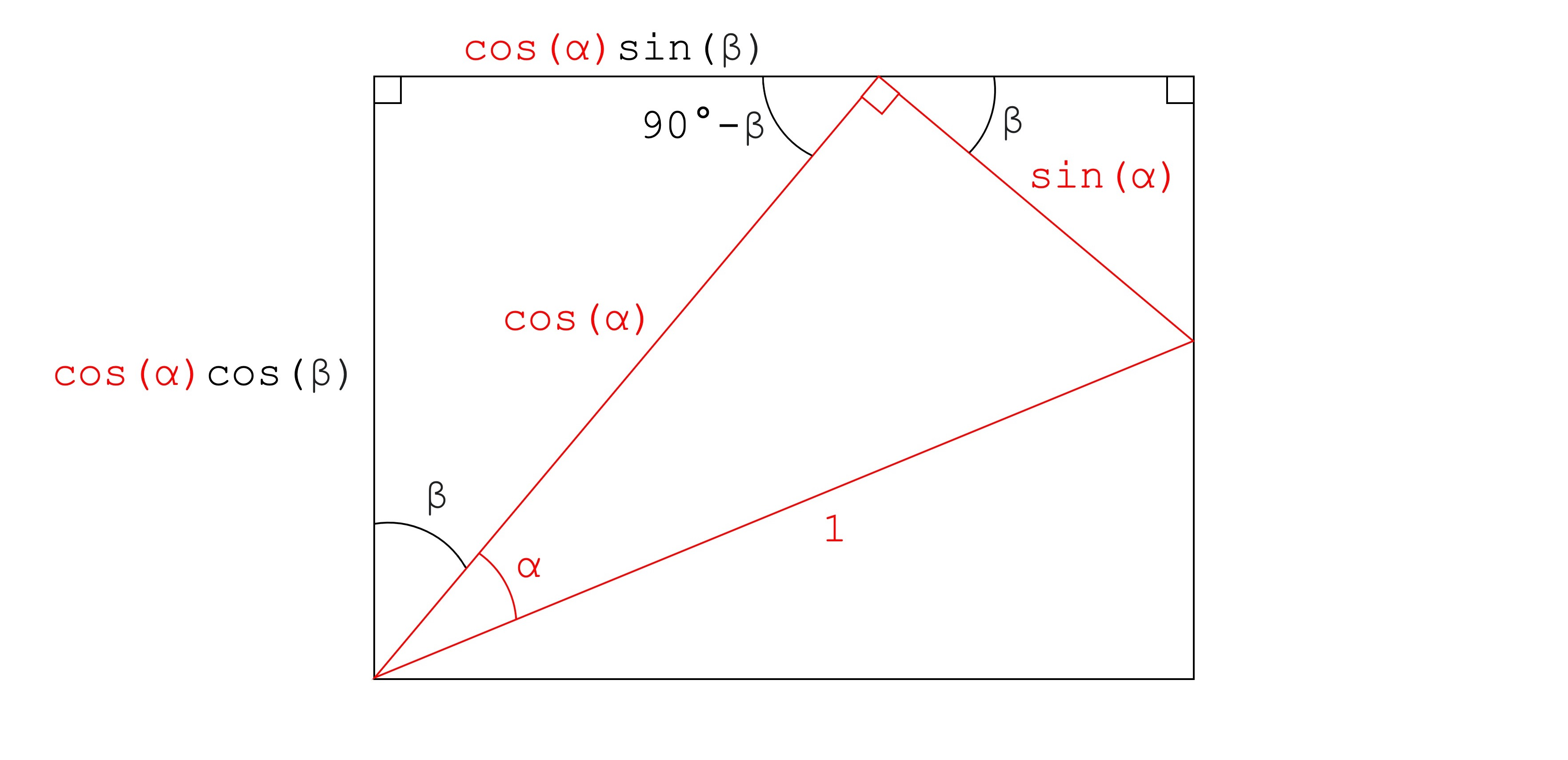### Step 5

With $\beta$ and the hypotenuse of the right-angled triangle on the top right, we can calculate the length of the opposite and adjacent sides using the definitions of sine and cosine with the angle $\beta$ and having a hypotenuse of length $sin(\beta)$.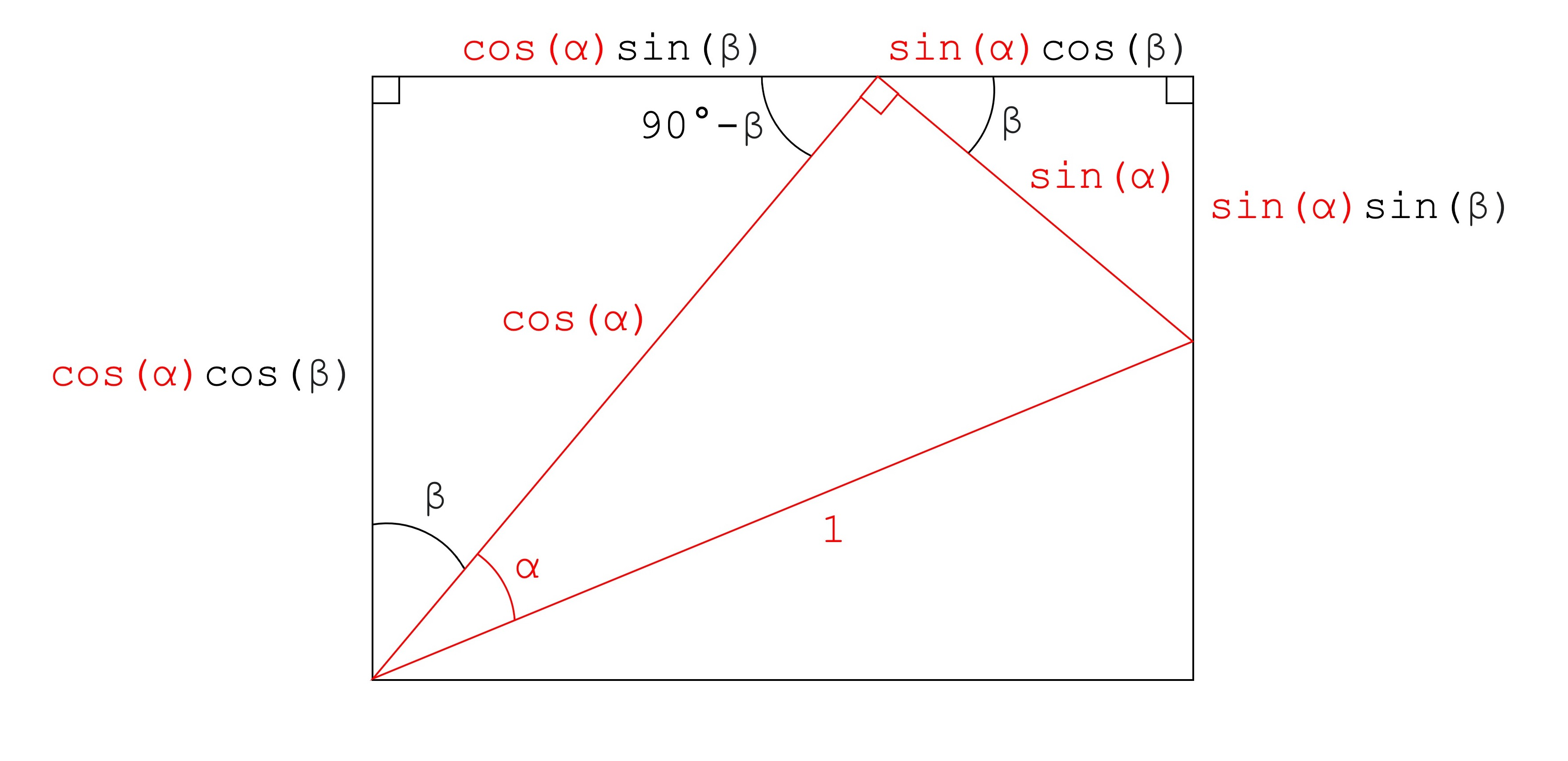### Step 6

There are only two steps remaining to reach the goal of obtaining the lengths of all sides of the rectangle. Moreover, The right-angled triangle at the bottom will give use the remaining lengths.

Given that the bottom left corner of the triangle forms an angle of 90°, we can define the angle corresponding to the corner of the bottom triangle as $90°-\alpha-\beta$.

Having the right angle and the angle described above, the remaining angle of the bottom triangle is equal to $180°-90°-(90°-\alpha-\beta)=\alpha+\beta$.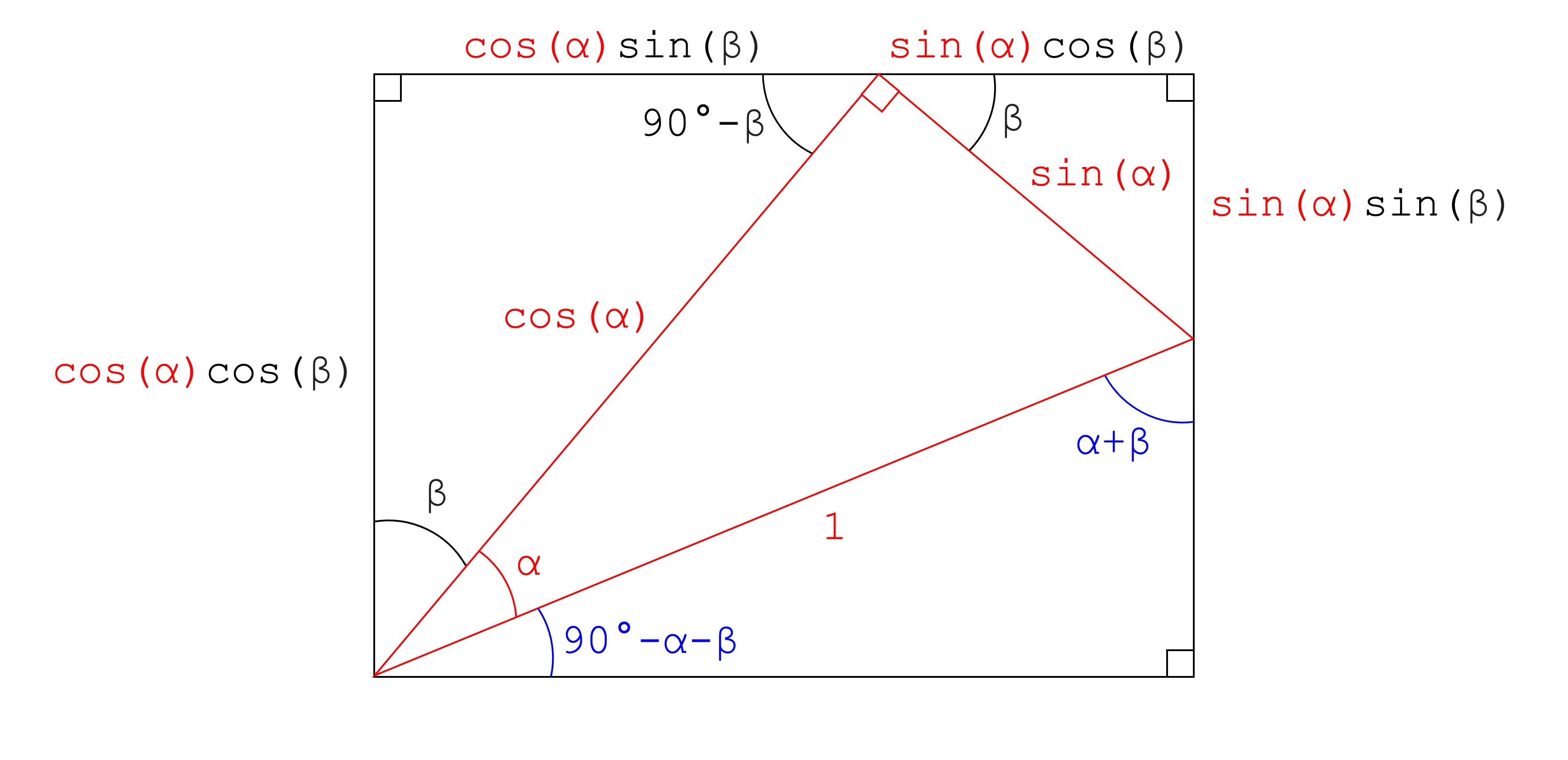### Step 7

Now we can find the remaining lengths of the sides of the rectangle by calculating the opposite and adjacent sides of the bottom triangle using the definitions of sine and cosine with the angle $\alpha + \beta$.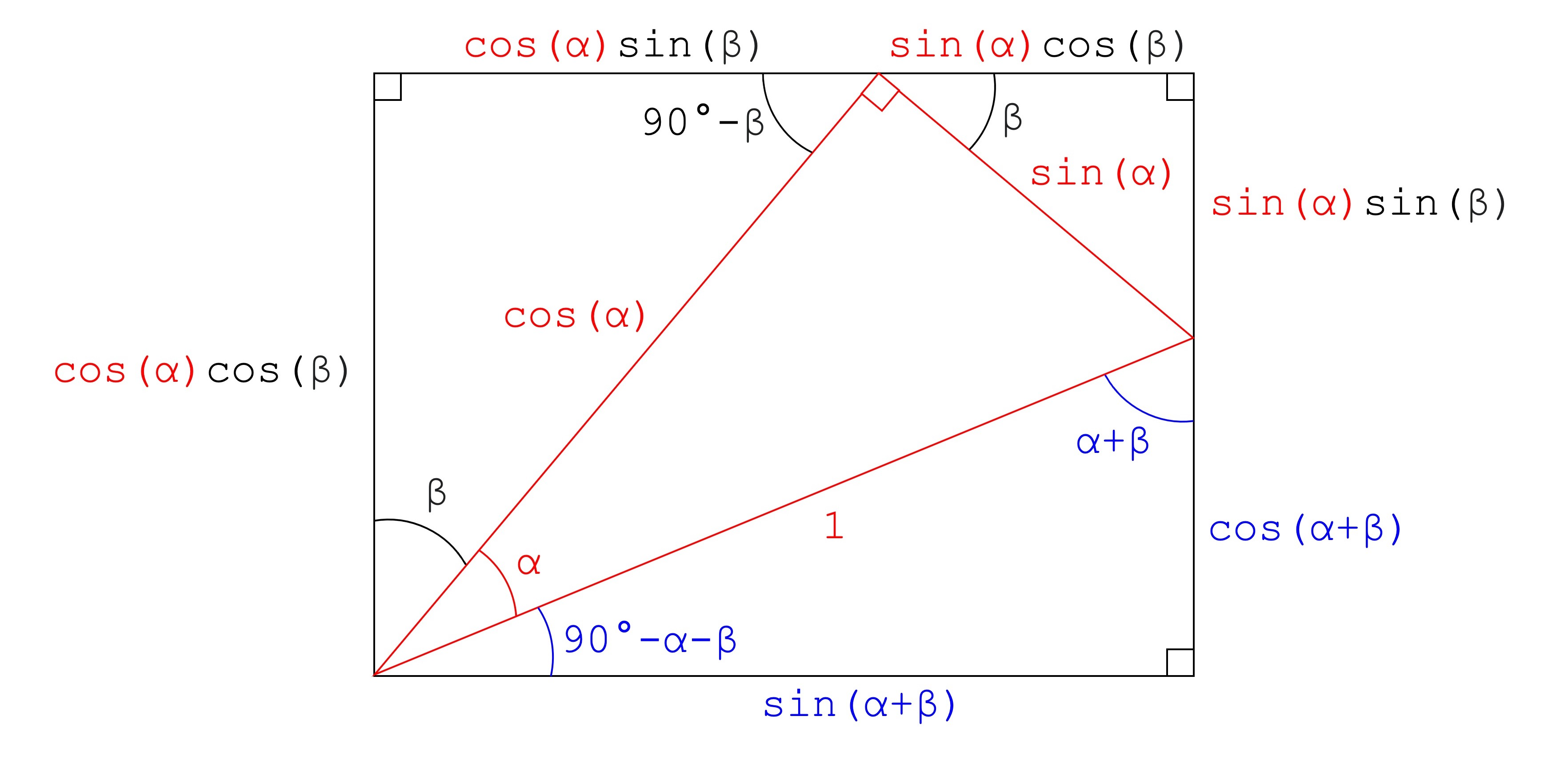### Step 8

Having found the total lengths of all four sides of the rectangle, we can use the fact that parallel sides have the same length to draw the following equivalences.

Equating the top and bottom sides:
$cos(\alpha)sin(\beta)+sin(\alpha)cos(\beta)=sin(\alpha+\beta)$

Equating the left and right sides:
$cos(\alpha)cos(\beta)=sin(\alpha)sin(\beta)+cos(\alpha+\beta)$

The equations above can be rewritten to reveal the angle sum and difference identities as shown earlier:

$sin(\alpha+\beta)=sin(\alpha)cos(\beta)+cos(\alpha)sin(\beta)$
$cos(\alpha+\beta)=cos(\alpha)cos(\beta)-sin(\alpha)sin(\beta)$

QED

## Wrapping up

Trigonometry is a fundamental topic on mathematics as well as a prerequisite to subjects like calculus, physics or linear algebra. Having a good understanding of the trigonometric identities allows us to identify when these relations can be used in scenarios where angles are involved, such as when working with vectors in space. Luckily it is relatively easy to visualise trigonometric relations as we can draw triangles and other basic geometric shapes which allows to obtain intuition by using visual proofs.

## External resources

1. I highly recommend watching this excellent video from the YouTube account blackpenredpen where the instructor goes through this proof step by step on a whiteboard.
2. Additionally the Wikipedia article about trigonometric identities is a good place to learn about the angle sum and difference, as well as other identities in more detail.